# AGNI – Avagadro’s Gravity for Nuclear Interactions

By

U.V.S. Seshavatharam
DIP QA Engineer, Lanco Industries Ltd, Srikalahasti-517641, A.P, India
E-mail: seshavatharam.uvs@gmail.com

Prof. S. LAKSHMINARAYANA
Department Of Nuclear Physics, Andhra University, Vizag-530003, AP, India.
E-mail: lnsrirama@yahoo.com

Abstract 1:
`N’ being the Avagadro number, it is suggested [2, 3] that strong nuclear gravitational constant (GS) is N2 times the classical gravitational constant (GC). Elementary charge (e) and the proposed (GS) plays a vital role in strong interaction and nuclear space-time curvature. Considering the present signi cance of Avagadro number  it is clear that, existence of the classical gravitational constant (GC) is a consequence of the existence of the strong nuclear gravitational constant (GS). It is also suggested that there exists 2 kinds of mass units. They can be called as `observed mass units’ and `hidden mass units’.
XE ≈ 295.0606339 being the lepton mass generator [1, 2, 3] hidden mass unit is XE times smaller than the observed mass unit. This idea can be applied to leptons and all the strongly interacting particles. For electron its hidden mass unit is 3.087292 x 10^-33 Kg.
Hidden mass unit of the previously proposed [1, 2, 3] strongly interacting fermion (MSf c^2 ≈ 105.3255407 MeV) is   MSf c^2/XE ≈ 0.35696236 MeV.
XE, the strong interaction mass generator = XS ≈ 8.803723452 and 0.35696236 MeV plays a vital role in understanding and coupling the semi empirical mass formula with TOE . ( αXE) is the ratio of coulomb energy coefficient (Ec) and the proposed (MSf c^2/XE) .
Proton and neutron rest masses are co related in a uni ed approach. GS, hidden mass units of electron and super symmetric  (MSf ) play a vital role in the origin of ћ.
Electron`s discrete angular momentum is due the strong nuclear gravity and discrete number of super symmetric nucleons or (MSf). All these coincidences clearly suggest that existence of the strong nuclear gravitational constant (GS) and existence of the strongly interacting fermion (MSf c^2) ≈ 105.3255 MeV are true and real.

 U. V. S. Seshavatharam and S. Lakshminarayana. Super Symmetry in Strong and Weak interactions. IJMPE, Vol.19, No.2, (2010), p.263-280.

 U. V. S. Seshavatharam and S. Lakshminarayana. Strong nuclear gravitational constant and the origin of nuclear planck scale. Progress in Physics, vol. 3, July, 2010, p. 31-38.

 U. V. S. Seshavatharam and S. Lakshminarayana. Avagadro number and the mystery of TOE and Quantum Theory. Under review of Journal of Nuclear Physics, Italy. (Old version is accepted for publication).

Abstract 2:
`N’ being the Avagadro number, it is suggested that there exists a charged lepton mass unit mL = 3.087292 x 10^-33 Kg in such way a that its electromagnetic and classical gravitational force ratio is N^2. Assuming that N neutrons transforms into 1/2N neutrons, 1/2N protons and 1/2N electrons a simple relation is proposed in between the lepton mass generator XE [1,2] strong gravitational constant GS , classical gravitational constant GC and the Avagadro number N.  XE being the proportionality ratio electron rest mass is proportional to its charge e and inversely proportional to N and √GC.
Muon and tau rest masses are tted. With a new (uncertain) quantum number at n=3, a new heavy charged lepton at 42260 MeV is predicted.
Considering N, 2N, 3N… moles XE takes discrete values and it can be shown that ћ is a true uni ed compound physical constant. A simple relation is proposed for estimating  the mass of strong interaction mass unit MSf c^2 ≈ 105.398 MeV. From super symmetry  considering the proposed value of fermion-boson mass ratio = ψ = 2.2623411 values of nuclear stability factor Sf and strong interaction mass generator XS are revised in a unifi ed manner.
Proton and neutron rest masses are tted to 4 decimal places. It it is suggested that XE sin(θw) ≈ 1/α . Finally in sub quark physics  the proposed strongly interacting fermionic mass unit 11450 MeV is tted with ln (N^2).

PACS numbers: 12.10-g,21.30.-x.

Keywords: true grand uni cation, classical gravitational constant, strong nuclear gravitational constant, lepton mass generator, characteristic lepton mass unit, leptons rest mass, new heavy charged lepton, strong interaction mass generator, proton and neutron rest masses, strong nuclear fermion, strong nuclear sub quark fermion, fermion-boson mass ratio 2.26.
.
1. Introduction
In this paper previuosly [1, 2] de ned lepton mass generator XE is rede ned in a uni ed approach and is shown that it is more fundamental than the ne structure rartio α.
Muon and Tau masses are fi tted. With a new (uncertain) quantum number at n=3, a new heavy charged lepton is predicted at 42260 MeV. Without considering the classical gravitational constant GC establishing a relation in between charged particle’s mass and charge is impossible. Till now Avagadro number  is a mystery. The basic counting unit in chemistry, the mole, has a special name Avogadro’s number in honor of the Italian scientist Amadeo Avogadro (1776-1856). The commonly accepted de nition of Avogadro number is the number of atoms in exactly 12 g of the isotope 6C12 and the
quantity itself is 6.02214199(47)10^23. Considering N as a fundamental input in grand uni ed scheme authors made an attempt to corelate the electron rest mass and its charge.
It is also noticed that h is slipping from the net and there lies the the secret of true grand uni fication.
As the culmination of his life work, Einstein wished to see a uni cation of gravity and electromagnetism  as aspects of one single force. In modern language he wished to unite electric charge with the gravitational charge (mass) into one single entity.
Further, having shown that mass the gravitational charge was connected with space-time curvature, he hoped that the electric charge would likewise be so connected with some other geometrical property of space-time structure. For Einstein [5, 6] the existence, the mass, the charge of the electron and the proton the only elementary particles recognized
back in 1920s were arbitrary features. One of the main goals of a uni ed theory should explain the existence and calculate the properties of matter.
Stephen Hawking – in his famous book- “A brief history of time”  says: It would be very difficult to construct a complete uni ed theory of everything in the universe all at one go. So instead we have made progress by nding partial theories that describe a limited range of happenings and by neglecting other e ects or approximating them by certain numbers. (Chemistry, for example, allows us to calculate the interactions of atoms, without knowing the internal structure of an atoms nucleus.) Ultimately, however, one would hope to nd a complete, consistent, uni ed theory that would include all these partial theories as approximations, and that did not need to be adjusted to fit
the facts by picking the values of certain arbitrary numbers in the theory. The quest for such a theory is known as “the uni cation of physics”. Einstein spent most of his later years unsuccessfully searching for a uni ed theory, but the time was not ripe: there were partial theories for gravity and the electromagnetic force, but very little was known about the nuclear forces. Moreover, Einstein refused to believe in the reality of quantum mechanics, despite the important role he had played in its development.
.
1.1. Charge-mass uni cation
The first step in uni fication is to understand the origin of the rest mass of a
charged elementary particle. Second step is to understand the combined e ffects of its electromagnetic (or charged) and gravatational interactions.Third step is to understand its behaviour with surroundings when it is created. Fourth step is to understand its behaviour with cosmic spce-time or other particles. Right from its birth to death, in all these steps the underlying fact is that whether it is a strongly interacting particle or weakly interacting particle, it is having some rest mass. To undestand the first 2 steps some how one must implement the gravitational constant in sub atomic physics. Till now quantitatively or qualitatively either the large number hypothesis or the string theory or the planck scale is not implemented in particle physics.
Unifying gravity with the other three interactions would form a theory of everything (TOE), rather than a GUT. As of 2009, there is still no hard evidence that nature is described by a Grand Uni ed Theory. Moreover, since the Higgs particle has not yet been observed, the smaller electroweak uni cation is still pending.The discovery of neutrino oscillations indicates that the Standard Model is incomplete. The gauge coupling strengths of QCD, the weak interaction and hypercharge seem to meet at a common length scale called the GUT scale and approximately equal to 1016 GeV, which
is slightly suggestive. This interesting numerical observation is called the gauge coupling unification, and it works particularly well if one assumes the existence of superpartners of the Standard Model particles.
.
1.2. Super symmetry
In particle physics, a superpartner (also sparticle) is a hypothetical elementary particle.
Authors proposed and clearly shown that in strong interaction there exists super symmetry  with a fermion-boson mass ratio, ψ ≈ 2.26 ( but not unity).  The word superpartner is a portmanteau of the words supersymmetry and partner. Supersymmetry is one of the synergistic bleeding-edge theories in current high-energy physics which predicts the existence of these “shadow” particles. According to the theory, each fermion
should have a partner boson, the fermion’s superpartner and each boson should have a partner fermion. When the more familiar leptons, photons, and quarks were produced in the Big Bang, each one was accompanied by a matching sparticle: sleptons, photinos and squarks. This state of a airs occurred at a time when the universe was undergoing a rapid phase change, and theorists believe this state of a airs lasted only some 10^-35 seconds before the particles we see now “condensed” out and froze into space-time.
Sparticles have not existed naturally since that time. In this case also authors shown that  these sparticles or super symmetric bosons can be seen at any time in the laboratory.
Boson corresponding to nucleon mass is 415 MeV and considering the basic idea of string theory that elementary particle masses are excited states of basic levels, it is clearly shown that 493, 547 and 890 MeV etc strange mesons are the excited states of 415 MeV boson. In the same paper  it is suggested that charged W boson is the super symmetric boson of Top quark! Finally authors wish to say that there is something wrong with the basic concepts of SM. After all from true grand uni cation point of view there is no independent existence for SM.
.
1.3. Planck mass, neutrino mass and Avagadro number
It is noticed that ratio of planck mass and electron mass is 2.389 x 10^22 and is 25.2 times smaller than the Avagadro number. Qualitatively this idea implements gravitational constant in particle physics. Note that planck mass is the heaviest mass and neutrino mass is the lightest mass in the known elementary particle mass spectrum. As the mass of neutrino is smaller than the electron mass, ratio of planck mass and neutrino mass will be close to the Avagadro number or crosses the Avagadro number. Since neutrino is
an electrically neutral particle if one is able to assume a charged particle close to neutrino mass it opens a window to understand the combined e ects of electromagnetic (or charged) and gravitational interactions in sub atomic physics. Compared to planck scale (past cosmic high energy scale), Avagadro number is having some physical significance in the (observed or present low energy scale) fundamental physics or chemistry.
.
2. Proposed new ideas in papers [1, 2]
In the previous papers [1, 2] authors collectively proposed the following new ideas.
1. Strong nuclear gravitational constant can be given as GS = 6.94273 x 10^31     m^3/kg sec^2.
2. There exists two strongly interacting “confined” fermionic mass units MSfc^2 = 105.38 MeV and MGfc^2 = 11450 MeV.
3. In super symmetry, for strong and weak interactions boson mass is equal to fermion mass/2.26234.
4. There exists integral charge quark bosons and boso-gluons.
5. There exists integral charge quark e ective fermions and e ective fermi-gluons.
6. No two fermions couples together to form a meson. Only bosons couples together to form a meson. Light quark bosons couples with e ective quark fermi gluons to form doublets and triplets.
7. Strong interaction mass generator = XS = 8.8034856 and it can be considered as the inverse of the strong coupling constant.
8. Lepton mass generator = XE = 294.8183 is a number. It plays a crucial role in particle and nuclear physics.
9. In the semi empirical mass formula ratio of “coulombic energy coefficient” and the proposed 105.383 MeV is equal to α. The coulombic energy constant = EC = 0.769 MeV.
10. The characteristic nucleon’s kinetic energy or sum of potential and kinetic enetrgies is close to the rest energy of electron.
.
2.1. Nuclear force and charge distribution radii
With reference to the assumed strong nuclear gravitational constant GS, if it assumedthat charectristic total energy of the nucleon in the nucleus is close to the rest energy of electron mec^2, the nuclear force is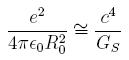and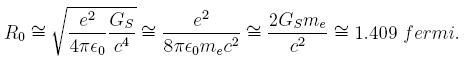Here, MSfc^2 is the assumed characteristic strong interaction fermionic mass unit=105.383 MeV, RC can be considered as the nuclear characteristic charge distribution radius.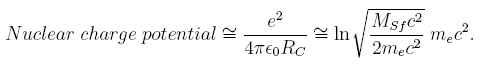.
Quantitatively to a very good accuracy it is noticed that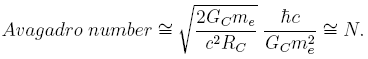Here, 2GCme/c^2 = classical Black hole radius of electron, RC = Nuclear charge radius ≈ 1.2157 fermi. This equation can be considered as a key observation for the implementation of Avgadro number in true uni cation. Using this equation value of the classical gravitational constant GC can be estimated accurately with the microscopic physical constants.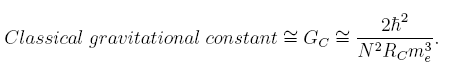On simpli cation eqation (5) can be written asWith the proposed lepton mass generator XE and the strong gravitational constant GS equation (7) can be simpli ed as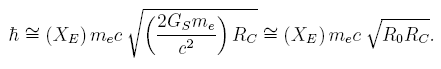Interestingly, 2GSme/c^2 ≈ strong nuclear black hole radius of electron ≈ 1:409 fermi ≈ R0, can be considered as the nuclear mass distribution radius. RC ≈ 1.2157 fermi is the nuclear charge distribution radius and geometric mean of RC and R0 is √R0RC ≈ 1.309 fermi. By considering “1 mole nucleons”, “2 mole nucleons”, “3 mole nucleons” etc XE takes “discrete” values like XE, 2XE, 3XE: Using this idea the origin of nh may be understood. Considering the nucleus-electron system, interestingly to a very good accuracy it is noticed that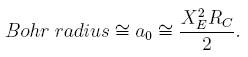Considering equations (3), (5), (17) and (22) all these observations can be obtained in a uni ed approach. Considering equation (29) the individual roles of MSf , GC and N in the nuclear physics can be understood. Considering equations (7) and (29) it can be suggested that, (N=2) represents a measure of uni ed gauge coupling strength.
.
3. Mole neutrons & relation between electron rest mass and its charge
Assuming that N neutrons transforms into 1/2N neutrons, 1/2N protons and 1/2N electrons authors tried to establish a relation in between the electron rest mass and its charge.
This idea may be a hypothesis or might have happened in the history of cosmic evolution.
For the time being authors request the world science community to consider this idea positively. Assume that out of N neutrons one neutron transforms into one proton and one electron. Focussing our attention to the rest energy of electron it is assumed that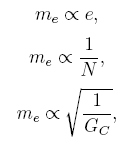On simpli cation it can be written asHere XE is a number and can be called as `lepton mass generator’ and EL =
1.732 x 10^-3 MeV can be called as the `characteristic lepton potential’.
Authors in the previous papers [1, 2 ] shown many applications of XE in particle
physics and nuclear physics. The weak coupling angle can be considered as (αXE )^-1 = sin(θw). It plays a crucial role in estimating the charged lepton rest masses. Ratio of Up and Down quark masses is αXE. It plays a very interesting role in tting energy coefficients of the semi empirical mass formula. It can be used for tting the nuclear size with “compton wavelength of nucleon”. It is noticed that ratio of “nuclear volume” and “A nucleons compton volume” is XE. It can be called as the nuclear “volume ratio” factor. In this paper in a uni ed approach XE is redi ned asHere, N/2= half mole neutrons or half mole protons or half mole electrons. GS = strong nuclear gravitational constant and GC = classical gravitational constant.
Till now Avagadro number is a mystery. The strange observation is, by considering “1 mole nucleons”, “2 mole nucleons”, “3 mole nucleons” etc XE takes “discrete” values like XE, 2XE, 3XE… Using this idea the origin of may be understood.
In equation (17) by seeing the strong gravitational constant GS every one will be surprised. But it is a fact. In the paper  authors proposed many applications of GS in nuclear physics. If neutron is a strongly interacting particle and its origin is related to GS it is reasonable and a must to implement GS in weak decay of neutron. Considering equation (17) electron rest mass can be given as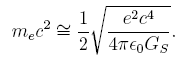In this way value of strong nuclear gravitational constant can be estimated from electron rest mass as GS ≈ 6.950631729 x 10^31     m^3/kgsec^2. From equation (15) XE can be obtained as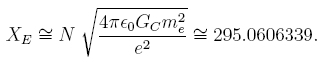If one is able to assume or guess that,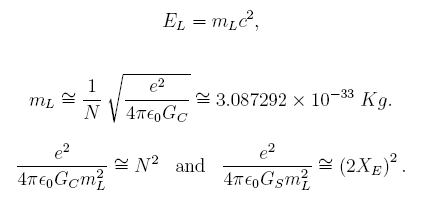From this idea it can be suggested that there exists a charged lepton mass unit
mL = 3.087292 x 10^-33 Kg in such way a that its electromagnetic and classical gravitational force ratio is N^2. It plays a crucial role in fi tting the rest masses of muon and tau.
.
4. Fitting of muon and tau rest masses
In the earlier paper  authors proposed the following relation for tting the muon and tau masses.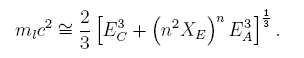Here EC= coulombic energy coefficient of the semi empirical mass formula =0.769 MeV, EA= assymmetry energy coefficient of the semi empirical mass formula=23.86 MeV and XE = proposed lepton mass generator = 294.8183 and n = 0, 1, 2. In this paper authors simpli ed equation (23) aswhere n=0,1 and 2 and EL ≈ 1.732 x 10^-3 MeV.
Equation (24) is free from all the binding energy coefficients of the semi-empirical mass formula. Authors hope that the eld experts can easily interpret this expression. See the following Table 1 .
If electron mass is tting at n=0, muon mass is tting at n=1 and tau mass is
tting at n=2 it is quite reasonable and natural to predict a new heavy charged lepton at n=3. Electron was discovered in 1897. Muon was discovered in 1937. Tau was detected in a series of experiments between 1974 and 1977. Positron predicted in 1928 and discovered in 1936. The antiproton and  antineutron were only postulated in 1931 and 1935 respectively and  discovered in 1956. The charged pion was postulated in 1935 and discovered in 1947 and the neutral pion was postulated in 1938 and discovered in 1950.
The 6 quarks were proposed and understood in between 1964 and 1977. By selecting the proper quantum mechanical rules if one is able to con rm the existence of the number n=3, existence of the new lepton can be understood.
At the same time one must critically examine the propsed relation for its nice and accurate tting of the 3 observed charged leptons. Unfortunately inputs of this expression are new for the standard model. Hence one can not easily incorporate this expression in standard model. Till now in SM there is no formula for tting the lepton masses accurately. It seems there is something missing from the SM. Not only that the basic inputs of SM are leptons and quarks. Now in this propsed expression authors tried to t and understand the origin of the fundamental building blocks of electromagnetic interaction! Same authors tried to t and understand the origin of quarks in the paper  with the electron rest mass as a reference mass unit. More interesting thing is that in the paper  authors proposed the existence of `integral charge’ qaurk fermions and`integral charge’ quark bosons. Eventhough this idea is against to the SM, observed strong interaction particles charge-mass spectrum can be understood very easily. Super symmetry plays a key role in this.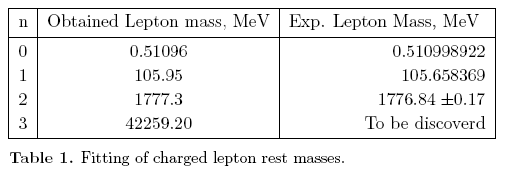5. Strong interaction mass unit MSf c^2
In paper  it is proposed that there exists a strongly interacting fermionic mass unit MSf c^2 ≈ 105.38 MeV. It is noticed that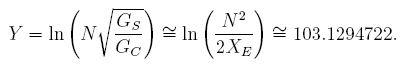Accuracy of MSf c^2 depends on XE and GS.6. Proton, neutron rest masses & the nuclear stability factor (Sf )
Qualitatively and quantitatively with 99.9 % accuracy it is noticed that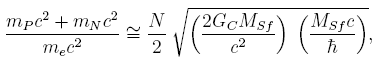where mP c^2 = rest energy of proton, mNc^2 = rest energy of neutron, mec^2 = rest energy of electron and MSf = proposed strong interaction fermion mass unit ≈ 105.398 MeV. Interesting thing is that, 2GCMSf/c^2 can be considered as the classical black hole radius of MSf and ћ/MSf c is the compton length of MSf . This equation clearly suggests the individual roles of MSf , GC and N in the nuclear physics. On simpli cation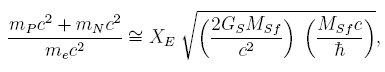Here, 2GSMSf/c^2 can be considered as the strong nuclear black hole radius of MSf . Considering the average mass of nucleonFrom equation (18)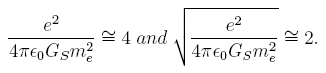Hence, in the above equation (31) denominator 2 can be eleminated asHere, e^2/4πεθmec is the classical radius of electron. On simpli cation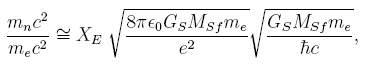All these equations suggests that the proposed strong nuclear gravitational constant GS and the strong interaction mass unit MSf plays a key role in understanding the origin of rest mass of nucleon.
.
6.1. Fitting of nucleon rest masses upto 4 decimal places
In paper  authors prosed a new number called as nuclear stability number Sf = 2XS^2 ≈ 155.0 and XS = Strong interaction mass generator ≈ √(GSM^2Sf/ћc). It is noticed that proportionality constant being √(1/Sf) ratio of rest energy of proton and charged lepton potential can be given aswhere mP c^2 = Rest energy of proton, EL = characteristic charged lepton potential ≈ 1.732 x 10^-3 MeV.Considering proton-electron mass ratio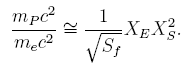If Sf is fundamental compared to XS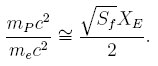This equation can be written as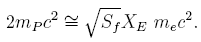If it is assumed that (mP c^2 + mNc^2) is more appropriate than 2mP c^2,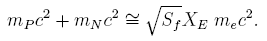Neutron-proton mass di erence can be given asSee the reference . In super symmetry in strong and weak interactions for every fermion there exists a corresponding boson of mass = (fermion mass/Ψ). Authors clearly shown this in paper . The most interesting idea is that fermion makes 13 jumps in one revolution and comes to the initial position and boson makes 13 revolutions with 6 jumps in each incomplete revolution. Here angle of jump for fermion can be assumed as sin^-1(1/αXE)≈27.67394^0 and angle of jump for boson is 55.34788^0. Boson in one cycle makes 78 jumps and this is very close to XS^2≈77.6.
Considering decay it is known that neutron transforms into stable proton, stable electron and stable neutrino. Authors proposed that neutron is a combination of proton and Up quark boson . The Up boson transforms into an electron. The important observation is that ψ plays a crucial role in neutron and proton mass generation. Its square root value is just crossing 1.5. This number 1.5 can also be attributed to the energy ratio of proposed  nuclear coulombic energy constant 0.769 MeV and the electron rest mass 0.511 MeV. But it is also not giving the correct values of mP and mN. Values of
mP and mN depends on Sf, XE and me. To a very good accuracy it is noticed that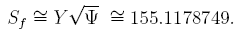where ψ = proposed strong interaction fermion – boson mass ratio – 2.2623412 ≈ ln(6+√13) and √ψ = 1.504108104.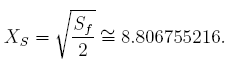This value of XS is very close to the original de nition of XS = √(GSMSf^2/ћc) = 8.809788028.
The obtained values are mP = 1.672641849 x 10^-27 Kg and mN = 1.67493932 x 10^-27 Kg. The co-data recommended  values are mP = 1.672621637 x 10^-27 Kg and mN = 1.674927211 x 10^-27.
.
6.2. Nucleon-proton stability
Stable isotope AS of any Z or proton-nucleon stability relation can be given asThis can be compared with the existing nucleon- proton stability relations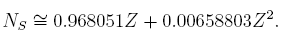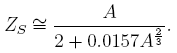Here NS=Stable neutron number, ZS=Stable proton number corresponding to mass number A. By considering A as the fundamental input its corresponding stable Z can be obtained as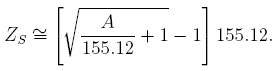AS can be called as the stable mass number of Z. After rounding o for even (Z)
values, if obtained AS is odd consider AS – 1, for odd (Z) values if obtained AS is even, consider AS – 1. For very light odd elements this seems to be not fitting. Hence for Z≤9 this correction idea is not applied. At Z = 47 obtained AS = 108.24. Its round o value is 108 which is even. Its nearest odd number is 108-1=107. At Z = 92 obtained AS = 238.56. Its round o value is 239 which is odd. Its nearest even number is 239-1=238. At Z = 29 obtained AS = 63.42. Its round o value is 63 which is odd. Correction is not required. At Z = 68 obtained AS = 165.81. Its round o value is 166 which is even. Correction is not required.
.
7. Estimation and signi cance of sin (θw)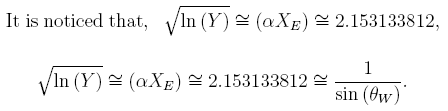Empirically it is noticed that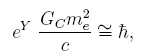Its more appropriate form seems to be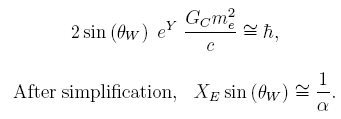8. Estimation of the strongly interacting subquark fermion MGf c^2 and the strongly interacting subquark boson MGbc^2
In the paper  it is proposed that there exists a strongly interacting fermionic mass unit MGf c^2 ≈ 11450 MeV in subquark physics by using which quark gluon masses can be estimated. It is noticed that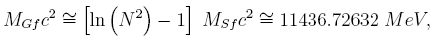It’s corresponding strongly interacting boson mass MGbc^2 can be given as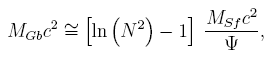where ψ =proposed strong interaction fermion-boson mass ratio=2.2623412.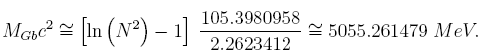Authors shown the applications of these two mass units in particle physics paper . MGf c^2 plays a crucial role in estimating the strongly interacting fermi-gluonic masses of e ective quark fermions as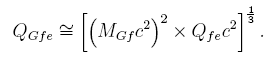where QGfe=e ffective quark fermi-gluon mass and Qfe=eff ective quark fermion mass. MGbc^2 plays a crucial role in estimating the strongly interacting boso-gluonic masses of quark bosons as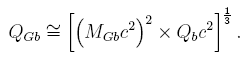where QGb=quark boso-gluon mass and Qb=quark boson mass.
.
Conclusions
If one is able to develop a relation between electron rest mass and charge certainly it can lead to the true grand uni cation. To understand the mystery of true grand uni cation Avagadro number can be given a chance. The characteristic nucleon’s kinetic energy or sum of potential and kinetic enetrgies is close to the rest energy of electron. Authors request the world science community to kindly look into these new ideas for further analysis.
.
Acknowledgements
Authors are very much grateful to the editors and referees of paper  and paper  for publication. First author is very much thankful to Prof. S. Lakshminarayana, Department of Nuclear Physics, Andhra University, Visakhapatnam, India, for his kind encouragement and guidance at all times. Finally rst author is very much thankful to his loving brother B. Vamsi Krishna (Software professional) for encouraging, providing technical and nancial support. Authors are very much thankful to the Wikipedia for the nice presenting of the `grand uni cation’ information.
References
 U. V. S. Seshavatharam and S. Lakshminarayana. Super Symmetry in Strong and Weak interactions. IJMPE, Vol.19, No.2, (2010), p.263-280.
 U. V. S. Seshavatharam and S. Lakshminarayana. Strong nuclear gravitational constant and the origin of nuclear planck scale. Progress in Physics, vol. 3, July, 2010, p. 31-38.
 Einsteins Last Dream: The Space -Time Uni cation of Fundamental Forces, Physics News, Vol.12, No.2 (June 1981), p.36. (Address by Professor Abdus Salam at the UNESCO Celebration of the Centenary of Einstein’s birth, 7 May 1979, Paris.)
 Tilman Sauer. Einsteins Uni ed Field Theory Program. The Cambridge Companion to Einstein, M. Janssen, C. Lehner (eds), Cambridge University Press.
 David Gross. Einstein and the search for Uni cation. http:worldscibooks.com/etextbook/6259.
 Hawking S.W. A Brief History of Time. Book. Bantam Dell Publishing Group. 1988.
 Particle Data Group (W.-M. Yao et al.), J. Phys. G 33 (2006) 1, http://pdg.bbb.gov.
 P.J. Mohr and B.N. Taylor. CODATA Recommended Values of the Fundamental Physical
Constants.2007. http://physics.nist.gov/constants.
 P. Roy Chowdhury et al. Modi ed Bethe-Weizsacker mass formula with isotonic shift and new driplines. (http://arxiv.org/abs/nucl-th/0405080v4)

• Andrea Rossi

Deborah Rivera:
Thank you from the whole Team,
Warm Regards,
A.R.

• Congratulations to you and your team: great work, we all are with you with our spirit! 🙂

• What a well crafted blog post you have right here. You actually managed to truly high light the important aspects that really matter. I really enjoy reading through your site, the quality of the data is superb and your writing style certainly makes it easy to digest and fully grasp. Fantastic! Keep up the good work.

• Andrea Rossi

We agree with you: AGNI is an important approach to through light on elementary particles.
A.R.

RESPECTED SIR,FINE APPROACH TO THROUGH LIGHT ON ELEMENTRY PARTICLES,QUANTA OF CHARGE THEIR UNIFICATION.THANKS FOR PROVIDING SO MUCH VALUABLE INFORMATION.

• finally found somewhere with useful details. thanks alot and keep it coming 🙂

• Andrea Rossi

Very interesting, also for possible theoretical declinations in the fusion models.
With the occasion we want to inform our Readers that Enrico Billi, an Italian Physic researcher from the University of Bologna who works in China, has sent us an important paper of his, under Peer reviewing at the moment, that probably will be published in January on the Journal Of Nuclear Physics.